Engineering Mechanics - PKRB: Force and Animation

Why Engineering Mechanics PKRB: Force and Animation?

In this section you can learn and practice Engineering Mechanics Questions based on "PKRB: Force and Animation" and improve your skills in order to face the interview, competitive examination and various entrance test (CAT, GATE, GRE, MAT, Bank Exam, Railway Exam etc.) with full confidence.

Where can I get Engineering Mechanics PKRB: Force and Animation questions and answers with explanation?

IndiaBIX provides you lots of fully solved Engineering Mechanics (PKRB: Force and Animation) questions and answers with Explanation. Solved examples with detailed answer description, explanation are given and it would be easy to understand. All students, freshers can download Engineering Mechanics PKRB: Force and Animation quiz questions with answers as PDF files and eBooks.

Where can I get Engineering Mechanics PKRB: Force and Animation Interview Questions and Answers (objective type, multiple choice)?

Here you can find objective type Engineering Mechanics PKRB: Force and Animation questions and answers for interview and entrance examination. Multiple choice and true or false type questions are also provided.

How to solve Engineering Mechanics PKRB: Force and Animation problems?

You can easily solve all kind of Engineering Mechanics questions based on PKRB: Force and Animation by practicing the objective type exercises given below, also get shortcut methods to solve Engineering Mechanics PKRB: Force and Animation problems.

Exercise :: PKRB: Force and Animation - General Questions

• PKRB: Force and Animation - General Questions
1.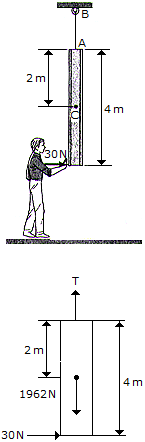The slender 200-kg beam is suspended by a cable at its end as shown. If a man pushes on its other end with a horizontal force of 30 N, determine the initial acceleration of its mass center G, the beam's angular acceleration, and the tension in the cable AB.

 A. aG = 0,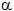= 0.225 rad/s2, T = 1.962 kN B. aG = 0.0750 m/s2,= 0.1125 rad/s2, T = 1.962 kN C. aG = 0,= 0.1125 rad/s2, T = 1.962 kN D. aG = 0.1500 m/s2,= 0.225 rad/s2, T = 1.962 kN

Explanation:

No answer description available for this question. Let us discuss.

2.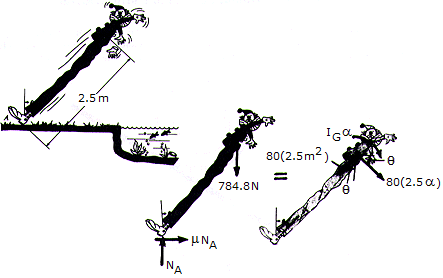A clown, mounted on stilts, loses his balance and falls backward from the position, where it is assumed the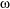= 0 when= 07deg;. Paralyzed with fear, he remains rigid as he falls. His mass including the stilts is 80 kg, the mass center is at G, and the radius of gyration about G is kG = 1.2 m. Determine the coefficient of friction between his shoes and the ground at A if it is observed that slipping occurs when= 30°.

 A.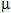= 0.833 B.= 0.468 C.= 0.243 D.= 0.400

Explanation:

No answer description available for this question. Let us discuss.

3.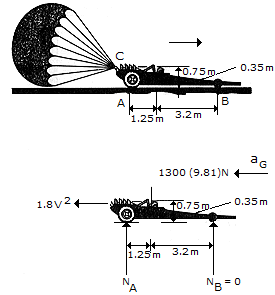The dragster has a mass of 1.3 Mg and a center of mass at G. If a braking parachute is attached at C and provides a horizontal braking force FD, determine the maximum deceleration the dragster can have upon releasing the parachute without tipping the dragster over backwards (i.e., the normal force under the wheels and assume that the engine is disengaged so that the wheels are freely rolling.

 A. a = 16.35 m/s2 B. a = 8.46 m/s2 C. a = 2.75 m/s2 D. a = 35.0 m/s2

Explanation:

No answer description available for this question. Let us discuss.

4.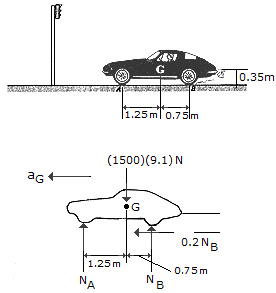The sports car has a mass of 1.5 Mg and a center of mass at G. Determine the shortest time it takes for it to reach a speed of 80 km/h, starting from rest, if the engine only drives the rear wheels, whereas the front wheels are free rolling. The coefficient of friction between the wheels and road is= 0.2. Neglect the mass of the wheels for the calculation.

 A. t = 17.49 s B. t = 18.12 s C. t = 18.76 s D. t = 22.7 s

Explanation:

No answer description available for this question. Let us discuss.

5.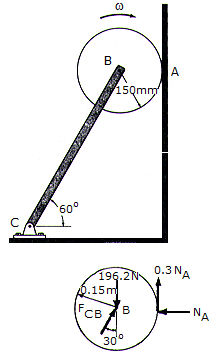The disk has a mass of 20 kg and is originally spinning at the end of the massless strut with an angular velocity of= 60 rad/s. If it is then placed against the wall, for whichA = 0.3, determine the time required for the motion to stop. What is the force in strut BC during this time?

 A. t = 3.11 s, FBC = 193.1 N B. t = 2.65 s, FBC = 227 N C. t = 5.30 s, FBC = 227 N D. t = 6.21 s, FBC = 193.1 N